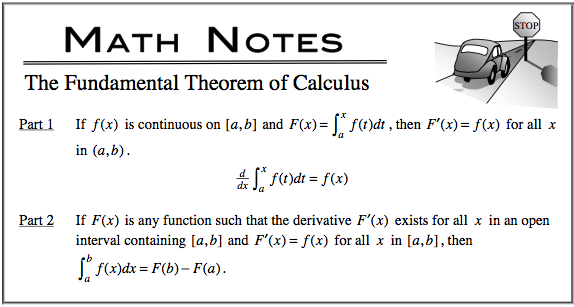### Home > CALC > Chapter 5 > Lesson 5.1.2 > Problem5-18

5-18.
1. Simplify each expression. Homework Help ✎

1.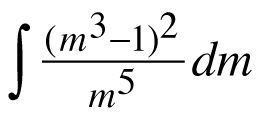2.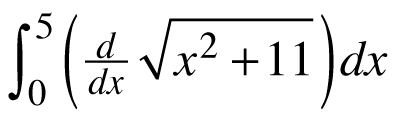3.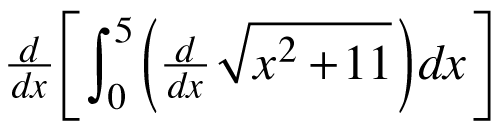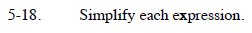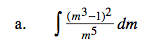1. Simplify the integrand by expanding the numerator and cancelling out the denominator.
2. Integrate by 'undoing' the Power Rule.
3. Don't forget the +C.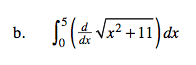Fundamental Theorem of Calculus (an extension):The integral of a derivative will give us the original function +C.

$6-\sqrt{11}$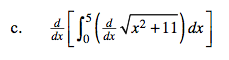$=\frac{d}{dx}[\text{part (b)}]$